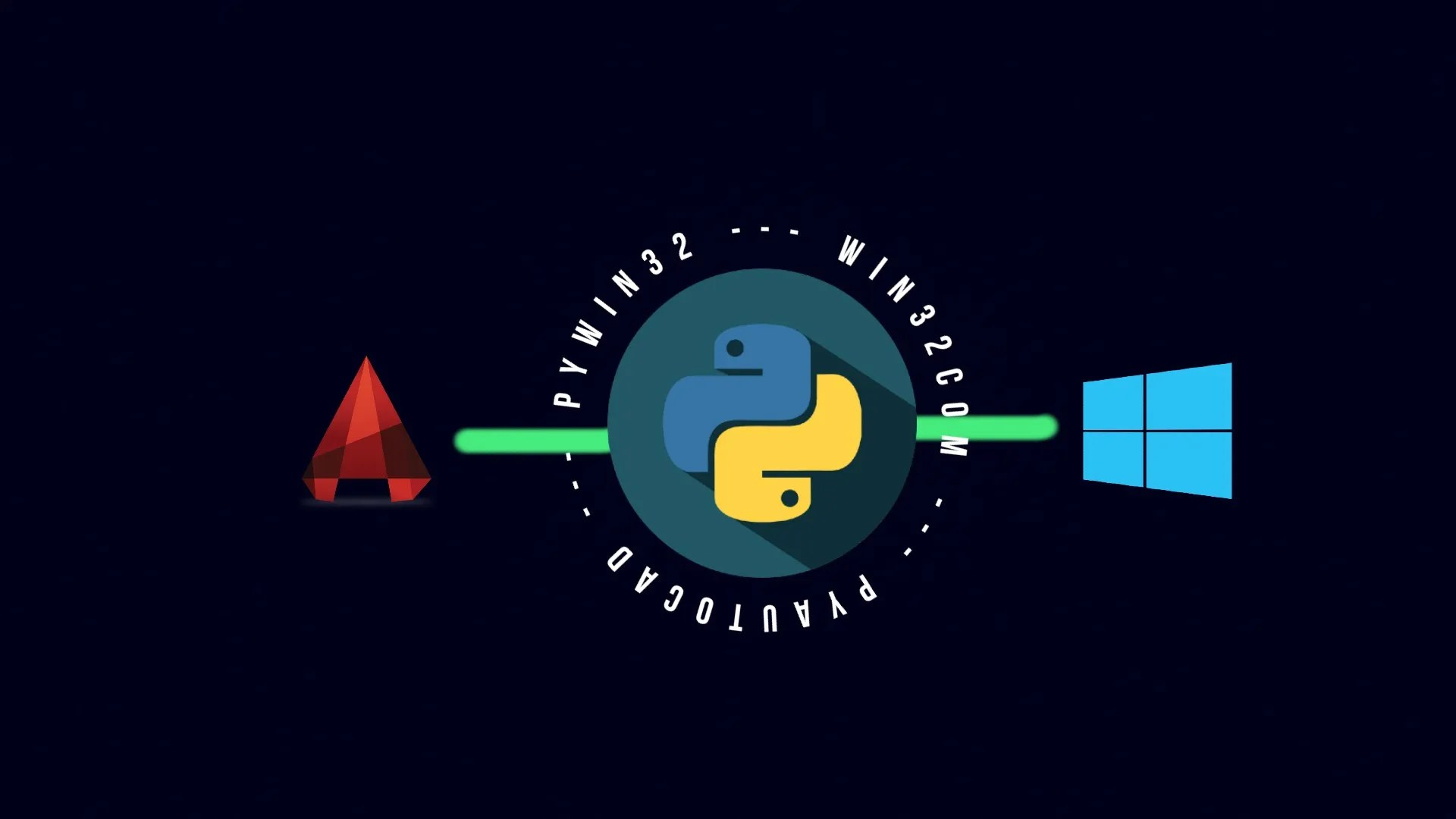# Using Python lists and dictionaries to work with AutoCAD objects with pyautocadNow that we have understood the basic usage of different pyautocad methods and objects from our previous blog posts we need to learn how to work with those more systematically and in an efficient way.

This blog post brings you a small solution for this.

## Use of Python lists and dictionaries for pyautocad objects

Sometimes the prebuild utilities aren’t enough for us.

Similarly, while using pyautocad, we want to group objects based on user-specified attributes or properties (i.e. object types) rather than existing prebuilt object types.

For instance, a civil engineer might want to separate columns, beams, footings and slab objects from one another in order for him or her to be able to deal with them separately.

In such a case we can use Python lists to store those objects separately, regardless of their geometrical similarities. And if we want to store objects while using their properties as their keys, we can use Python dictionaries.

## Use cases

As just discussed, let us create some objects and group them together using lists and dictionaries.

Here we are creating some random objects as given in the code below.

``````line1 = acad.model.AddLine(APoint(0, 0, 0), APoint(100, 100, 0))

pl1 = acad.model.AddPolyline(aDouble(0, 0, 0, 100, 0, 0, 100, 100, 0, 0, 100, 0, 0, 0, 0))
``````

Now, we’ll add these objects to a list and a dictionary, grouping them as per our requirements.

``````#Creating list for line objects
ls_line = []
ls_line.append(line1)
ls_line.append(line2)

#Creating dictionary for objects by specifying random properties as their keys
dict_mixed = {"round": circle1, "square": pl1}``````

Now, we’ll iterate through the objects in the list and will change their properties.

``````for l in ls_line:
l.ScaleEntity(APoint(l.StartPoint), 2)``````

Also, we can iterate through dictionary objects using keys as given below.

``````for j in dict_mixed:
if j == "round":
dict_mixed[j].ScaleEntity(APoint(dict_mixed[j].Center), 2)
elif j == "square":
dict_mixed[j].ScaleEntity(APoint(dict_mixed[j].Coordinate(4)), 2)``````

We can also filter objects based on the AutoCAD assigned property of value from a dictionary. For instance as in the demonstrated in the following lines of code:

``````for j in dict_mixed:
if dict_mixed[j].ObjectName == "AcDbCircle":
dict_mixed[j].ScaleEntity(APoint(dict_mixed[j].Center), 2)
elif dict_mixed[j].ObjectName == "AcDb2dPolyline":
dict_mixed[j].ScaleEntity(APoint(dict_mixed[j].Coordinate(4)), 2)``````